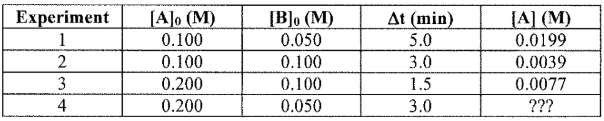# Problem: The following reaction is studied at 25 °C in benzene solution:CH3OH + (C6H5)3CCl → CH3OC(C6H5)3 + HCl   A                    BThe following experimental data have been collected:Based on the conditions provided in the table above, determine [A] for experiment 4.

###### FREE Expert Solution

We’re being asked to determine [A] for experiment 4.

CH3OH + (C6H5)3CCl → CH3OC(C6H5)3 + HCl

A                 B

Recall that the rate law only focuses on the reactant concentrations and has a general form of:

k = rate constant
A & B = reactants
x & y = reactant orders

We’re going to calculate the rate law and the rate constant using the following steps:

88% (93 ratings)###### Problem Details

The following reaction is studied at 25 °C in benzene solution:

CH3OH + (C6H5)3CCl → CH3OC(C6H5)3 + HCl

A                    B

The following experimental data have been collected:Based on the conditions provided in the table above, determine [A] for experiment 4.